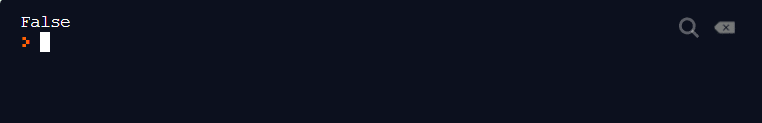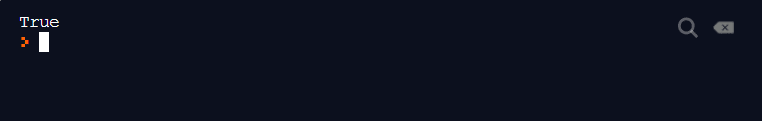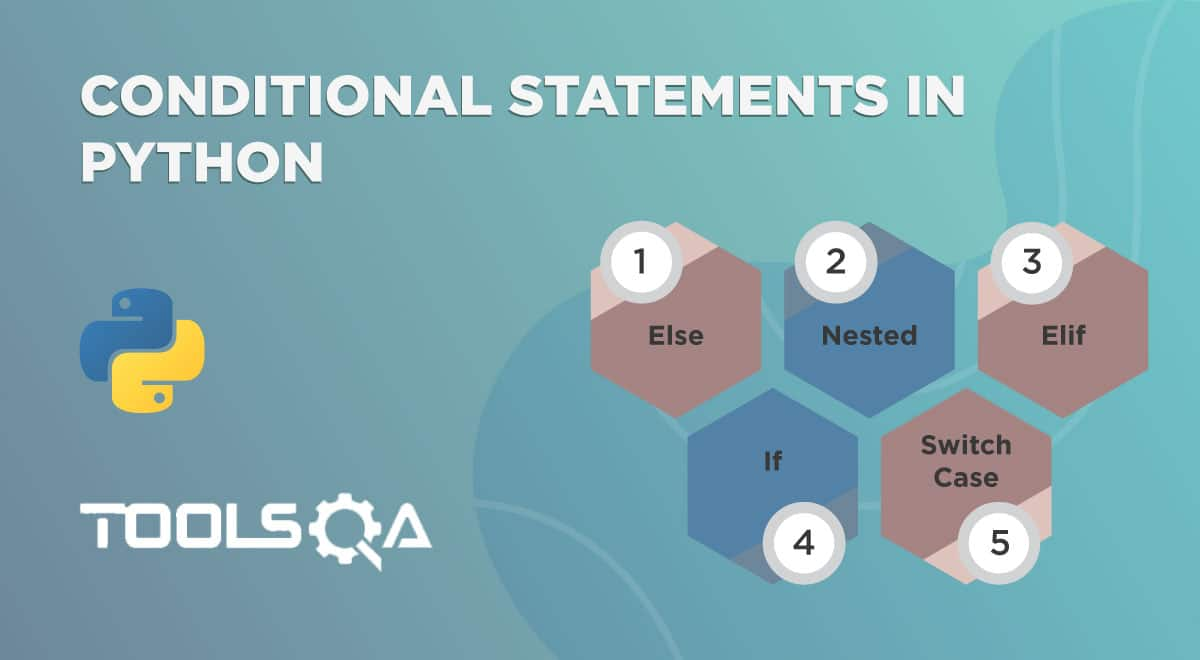In this course of PCEP, we have so far familiarized ourselves with the bitwise operators and boolean operators. Apart from these, we have another set of operators called Python comparison operators. They are widely used for comparing two operands. We will go through the below topics to learn more about these operators.

• What are python comparison operators?
• Python Comparison Operators
• Equal To Operator in python.
• Not Equal To Operator in python.
• Greater Than Operator in python.
• Less Than Operator in python.
• Greater Than Equal To Operator in python.
• Less Than Equal To Operator in python.
• Reference for python comparison operators.

## What are Python Comparison Operators?

Comparison operators in python do exactly what the name suggests: comparing. Comparison operators are also called relational operators as they find the relation between the operands (greater than, equal, etc.) and generate a boolean value in terms of True and False. These operators are used exhaustively from small to large programs in logic building and are therefore one of the most important concepts in the PCEP course. In this post, we will go through the six comparison operators in python and examine all of them with examples.

## Python Comparison Operators

Below is the list of six comparison operators used in Python.

### Equal To Operator in Python

The python equal to operator returns True if the two operands under consideration are equal to each other. Otherwise, the value returned is False. The "equal to" operator is denoted by two equal signs i.e. "==".

Execute the following code to see the output:

``````a = 2

b = 4

print(a == b)
``````As expected, since a is not equal to b (*with values 2 and 4 *), the answer returned is False.

### Not Equal To Operator in Python

The python not equal to operator returns True if the operands under consideration are not equal to each other. Otherwise, the answer returned is False. The "not equal to " operator is exactly opposite to the "equal to" operator in Python i.e. not(equal to) if it helps you remember better. The "not-equal-to" operator is denoted by "!=" sign.

Taking the same example as above, it should return True this time.

Execute the following code to see the output:

``````a = 2

b = 4

print(a != b)
``````And yes, it does return the expected answer on the console.

### Greater Than Operator in Python

One of the comparison operators in Python is the "greater than " operator. This operator is denoted by the symbol ">" and returns True if the operand on the left side has a greater value than the operand on the right side.

We will examine the same piece of code to see the result for a > b. Execute the following code:

``````a = 2

b = 4

print(a > b)

``````Since "***a ***" is less "b ", the answer returned on the console is False.

### Less Than Operator in Python

Contrary to the "greater-than " operator in Python, the less-than operator returns True if the operand on the left-hand side has a value lesser than the value of the right-side operand. Otherwise, the answer returned is False. The operator is denoted/ represented by the symbol "< ".

Execute the following code in the editor:

``````a = 2

b = 4

print(a < b)
``````Since the value of the operand "a " is less than the value of the operand "b ", the answer returned is True.

### Greater Than Equal To Operator in Python

The greater-than equal -to operator is the combination of two python comparison operators: equal-to and greater-than. The "greater than equal to " operator returns True if the value on the left right-side is either greater or equal to the value on the right side. This comparison operator is exactly similar to the greater-than operator with a condition that equal-to value is also considered during operation.

Execute the following code and check the answer in the console:

``````a = 10

b = 10

print(a >= b)
``````Since the values of operands a and b are equal, the answer returned is True. As a practice, execute the above code with just the "greater-than " operator and see the answer.

### Less Than Equal To Operator in Python

With the similar effects of the "greater than equal to " operator, the "the less than equal to " operator returns True if the operand value on the left side of the operator is either less-than or equal-to the operand value on the right side. This operator is a combination of "less-than " comparison operator and "equal-to " comparison operator. You can execute the same code as above to see the results without any changes.

## Reference for Python Comparison Operators:

I have designed a table for your quick reference to remember these operators and recall their working in a go.

Operator Description Example
Equal-To (== ) Decides whether the values are equal or not. a = 20, b = 20 a == b returns True
Not-Equal-To (!= ) Decides whether the values are unequal or not. a = 10, b = 12a != b returns True
Greater-Than (> ) Decides whether the left-value is greater or not. a = 25, b = 10 a > b returns True
Less-Than (< ) Decides whether the left-value if lesser or not. a = 10, b = 25 a < b returns True
Greater-Than-Equal-To (>= ) Decides whether the left-value is greater or equal to the right value or not. a = 10, b = 10 a >= b returns True
Less-Than-Equal-To (<= ) Decides whether the left-value is lesser or equal to the right value or not. a = 34, b = 34 a <= b returns True

In this post, we directly executed the comparison operators under the print statement to demonstrate more clearly how they work. However, in real programs, these operators are used for logic building under conditional statement along with the boolean operators (although not necessarily ). In the below section, I have written a small snippet to get you along the same tracks. But, you should keep practicing to get more comfortable yourself.

The following program helps avail the child benefit scheme to the government employees having a child younger than 20 years of age:

``````parent_occupation = "Government Emp"
number_of_children = 2
child1_age = 15
child2_age = 25
benefit = 0
if(parent_occupation == "Government Emp"):
if(number_of_children > 0):
if(child1_age <= 20):
benefit += 1000
if(child2_age <= 20):
benefit += 1000
else:
print("There is currently no policy for people with no children")
else:
print("This facility is only for government employees")
print("Total Benefit Availed :" + str(benefit))
``````

I hope this post was of help to you. This was all in the operator series in Python. You can visit the Boolean operators in Python and Bitwise operators in Python if you have not read them yet. This will build a strong foundation for you, and get you ready for upcoming topics.

## Key Takeaways:

• Comparison Operators in Python are used for comparing two operand values.
• Python comparison operators are also called as Python relational operators.
• The following are the python comparison operators:
• equal-to operator.
• not-equal-to operator.
• greater-than operator.
• less-than operator.
• greater-than-equal-to operator.
• less-then-equal-to operator.ReviewersLakshay Sharma